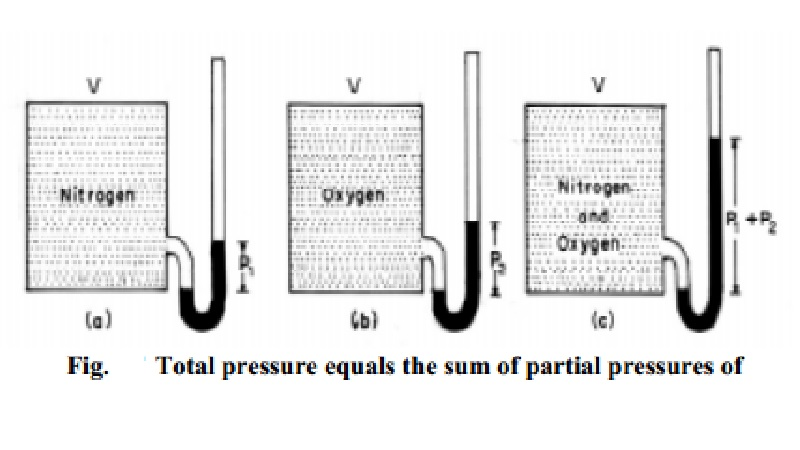Home | | Chemistry | | Chemistry | Numerical values of gas constant (R)

Numerical values of gas constant (R)The numerical value of the gas constant `R' depends upon the units in which pressure and volume are expressed, R = PV / T (assuming one mole of gas)

Numerical values of gas constant (R)

The numerical value of the gas constant `R' depends upon the units in which pressure and volume are expressed,

R  =            PV / T         (assuming one mole of gas)

R       = (Pressure x Volume) / Temperature

= (     Force / Area )  x ( Volume / Temperature )

Since Volume =    Area x length

R   = ( Force x Length ) /         Temperature

= Work / Temperature

The dimensions of R are thus energy per degree per mole.

i) In litre - atmosphere

One mole of gas at S.T.P occupies a volume of 22.4 litre and thus

At STP

P = 1 atm R = PV/T (for 1 mole)

V =    22.4 litre R =        (1 x 22.4)/273

T = 273 K        R = 0.0821 litre atm K-1mol-1

= 0.0821 dm3.atm K-1mol-1

( 1 litre = 1 dm3)

ii) In C.G.S. System

At STP 1 mole of gas has

P = 1 atm = 1 x 76 x 13.6 x 980 dyne cm-2

= 1.013 x 106 dyne cm-2

V =  22400 cm3; T = 273 K

R = PV / T  = 1.013 x 106 x 22400  / 273

R  =  8.314 x 107 erg K-1 mol-1

iii) In M.K.S. System

In MKS or SI units, the unit of R is joule

Since 107 erg        =       1 Joule

R = 8.314 Joule K-1 mol-1

1 calorie = 4.184 Joule

R = 1.987 cals deg-1 mol-1

Study Material, Lecturing Notes, Assignment, Reference, Wiki description explanation, brief detail

Related Topics